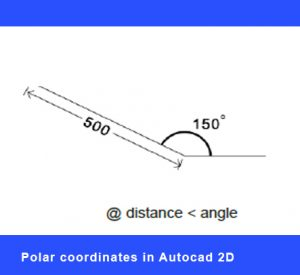Drawing using Polar Coordinates is a very useful feature when drafting in the Autocad 2D interface. This is one of the may functions which we explore at length in all of our Autocad 2D training courses.In previous blog posts we have looked at drawing using Absolute Coordinates, drawing using Dynamic Input, drawing using Relative Coordinates, and drawing using Polar Coordinates completes the overview of the various drafting methods employed in Autocad.

When working with Polar coordinates we establish both the distance and angle of a line, as follows. We can use the “at” (@) symbol on the keyboard to denote the distance and the “less than” symbol (<) to denote the angle. And this will draw the next point relative to to the last point drawn. In this way it’s similar to drawing using Relative Coordinates.

For example, in the diagram illustrated here we have first drawn a horizontal line. We then would type into the Command Line the following: @500<150, 500 being the distance in millimeters, and 150 being the angle as measured from the east (as if on a protractor).
Another way of looking  at it would be the formula @x<y from the previous point, or @dist<angle. Ending, as always, by hitting the Enter/Return key to complete the command.

In many ways this method of entering distances and angles has been largely superseded by the default use of Dynamic Input. In older versions of Autocad this was conducted by selecting the DYN button on the bottom Status bar. Doing so would allow the user to enter the line’s distance and angle at the cursor itself, and by pressing the Tab key to move from field to field. This is now the default setting within Autocad 2D, but it can, of course, be turned off via a system override.

More information on all features of the program can be found at the AutoDesk website. And see many examples of our clients’ work on our Facebook page.

Other related Autocad 2d Blog Posts: